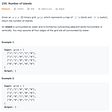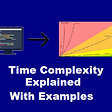# Introduction

In this article, we will learn how to implement a queue using an array in Javascript! A basic implementation of the queue data structure will be done with the methods:

1. enqueue() — Adds an element to the queue
2. dequeue() — Removes and returns the first item entered in the queue
3. isEmpty() — Returns true or false based on if the queue is empty or not
4. front() — Returns the front element of the queue
5. print() — Returns all the elements of the queue

Note: In my previous article, I discussed what the queue data structure is. Click here to read the article.

So let’s start!

# The simple way

You can implement a queue in Javascript pretty easily. For starters, let’s create an array.

`var queue = [];`

Next, let’s push some values using the push() method.

`var queue = [];queue.push(1);queue.push(2);`

Here we go! We have a basic queue ready, and we have completed the enqueue operation. Similarly, you can do the dequeue operation.

`queue.shift(); //dequeue operation`

To check whether the queue is empty, you can check whether the length of the array is empty or not.

`if(queue.length > 0){    console.log("The Queue is not empty")} else {     console.log("The Queue is empty") }`

You could even print the front element and print all elements.

`console.log(queue); //prints the front elementconsole.log(queue); //prints all the elements`

The above is a straightforward approach to queues. You can use this, but a better approach would be class-based.

# Class-based approach

## The node class

`class Node {    constructor(data) {       this.data = data;   }}`

The Node class will be used to create nodes which then can be enqueued or dequeued in the queue. The Node is an elementary class with just a constructor that initializes the object and assigns the data. This is a straightforward implementation, and you can add some customization to meet your requirements.

## The Queue class

Now, let’s create the main Queue class. This class will have these methods relating to the Queue data structure: enqueue(), dequeue(), isEmpty(), front(), print().

`class Queue {   constructor() {      this.elements = [];   }      enqueue(node) {      this.elements.push(node);   }    dequeue() {      if(this.elements.length > 0) {           return this.elements.shift();      } else {          return 'Underflow situation';      }   }   isEmpty() {      return this.elements.length == 0;   }      front() {      if(this.elements.length > 0) {          return this.elements;      } else {          return "The Queue is empty!";      }   }   print() {      return this.elements;   }}`

Now, let’s understand each part of the class Queue.

`constructor() {   this.elements = [];}`

The class again consists of a constructor which initializes the object. It also creates an empty array, which is used to create a queue.

`enqueue(node) {   this.elements.push(node);}`

The enqueue method (not a static method) enqueues the node (adds the node to the rear of the queue). At the base level, the node is pushed in the array. The push() method is available in javascript that pushes an element to the end of the array.

Note: The enqueue() method does not follow the overflow condition. The overflow condition checks whether the queue is full (has more memory available to add a new element). This overflow condition is not implemented in the class created by me, as we assume the queue is growing dynamically.

`dequeue() {  if(this.elements.length > 0) {        return this.elements.shift();  } else {       return 'Underflow situation';  }}`

The dequeue method (not a static method) dequeues/removes the element from the front of the queue. The method checks whether the elements in the queue are greater than 0; otherwise, the underflow situation occurs. At the base level, the element is shifted from the array. The shift() method is available in javascript that removes the first item from an array. Here is where the time complexity messes up. I have explained in my previous article: how the time complexity for the dequeue operation changes when implementing a queue using an array.

Note: The dequeue() method follows the underflow condition. The underflow condition checks whether the queue has any elements left for them to be dequeued. You cannot dequeue an empty queue, can you?

`isEmpty() {   return this.elements.length == 0;}`

The isEmpty method (none of the methods are static) checks whether the queue is empty or not. At the base level, it returns whether the length of the array is 0 or not. Well, this method was self-explanatory.

`front() {  if(this.elements.length > 0) {      return this.elements;  } else {      return "The Queue is empty!";  }}`

The front method returns the first element of the queue. It checks whether the elements array's length is greater than 0 and, based on that returns a value. You can customize the if-else statement to a shorter statement like ternary operators. I like the original way!

`print() {    return this.elements;}`

The final method, print() returns the elements array. Again, pretty self-explanatory.

# Does it work?

Now, let’s test if the queue is working. For that, I created a simple test code.

`const queue = new Queue();//Logs: trueconsole.log(queue.isEmpty());queue.enqueue(new Node({"number": 1}));queue.enqueue(new Node({"number": 2}));queue.enqueue(new Node({"number": 3}));queue.enqueue(new Node({"number": 4}));queue.enqueue(new Node({"number": 5}));//Logs: falseconsole.log(queue.isEmpty());/* Logs:[     Node { data: { number: 1 } },     Node { data: { number: 2 } },     Node { data: { number: 3 } },     Node { data: { number: 4 } },     Node { data: { number: 5 } }]*/console.log(queue.print());queue.dequeue();//Logs: falseconsole.log(queue.isEmpty());queue.dequeue();queue.dequeue();queue.dequeue();queue.dequeue();//Logs: trueconsole.log(queue.isEmpty());//Logs: []console.log(queue.print());`

# Final code

Here is the final code with the GitHub repository. Link: https://github.com/Megh-Agarwal/Queue-data-structure

We successfully implemented the queue data structure using an array in javascript! Congratulations! If you liked the article, a clap would motivate me to continue writing such articles :)

--

--

--

## More from Nerd For Tech

NFT is an Educational Media House. Our mission is to bring the invaluable knowledge and experiences of experts from all over the world to the novice. To know more about us, visit https://www.nerdfortech.org/.

## JS => or arrow functions## JAVASCRIPT AND ITS IMPORTANCE## Semantic UI, Make Your Website Pretty## Embedding AngularJS and React Like a Pro## How to Build Vue Components like a Pro 😎## Angular vs React what to choose in 2020?## Megh Agarwal

Incoming freshman at the University of Toronto. Founder, developer, designer of Pustakdaan. Experienced web developer. Interested in research (AI, ML).

## Number of Islands: Leetcode — Blind 75 (Graph)## Java Algorithms: Copy List with Random Pointer (LeetCode)## Time Complexity of Algorithms Explained with Examples